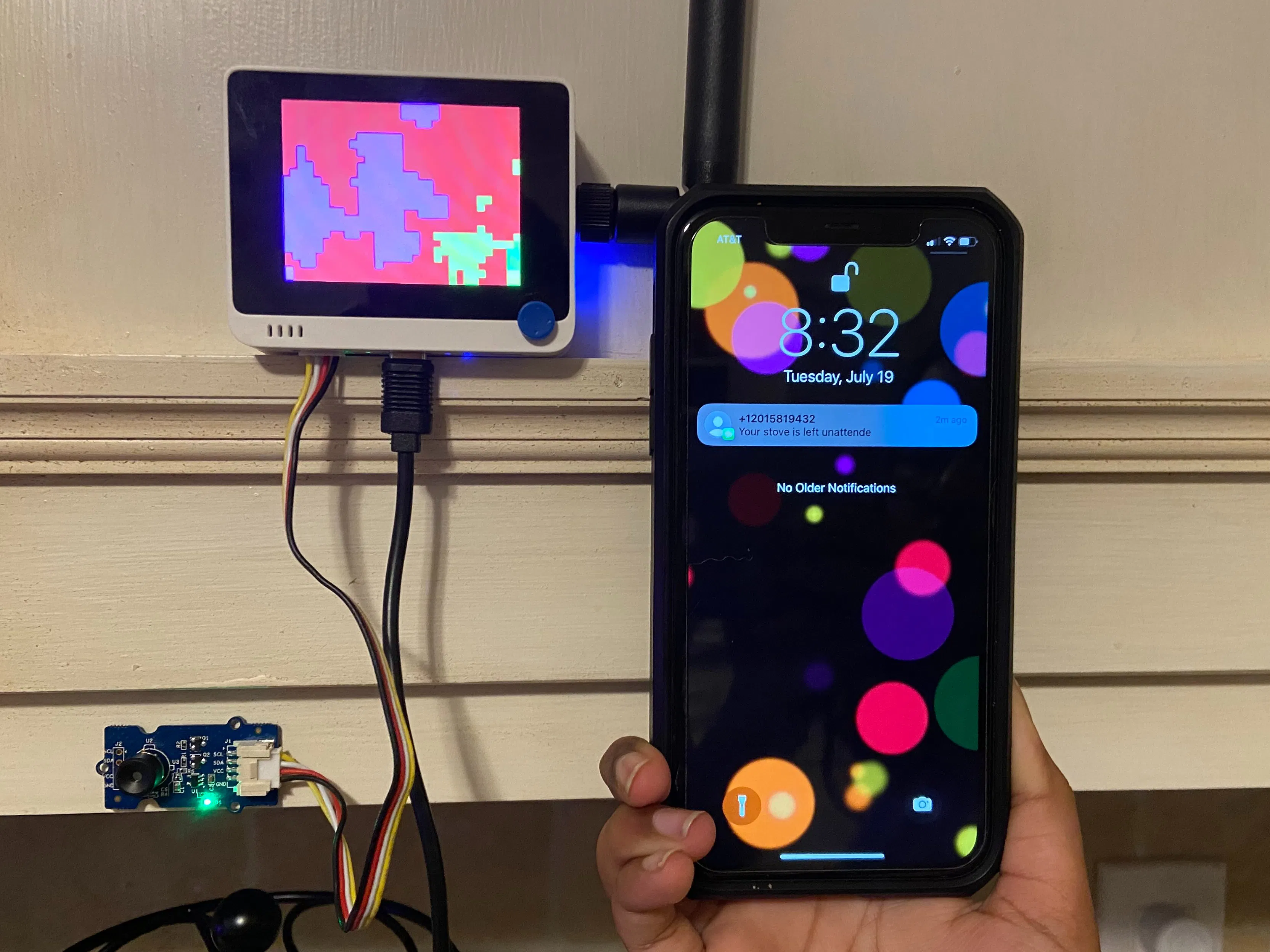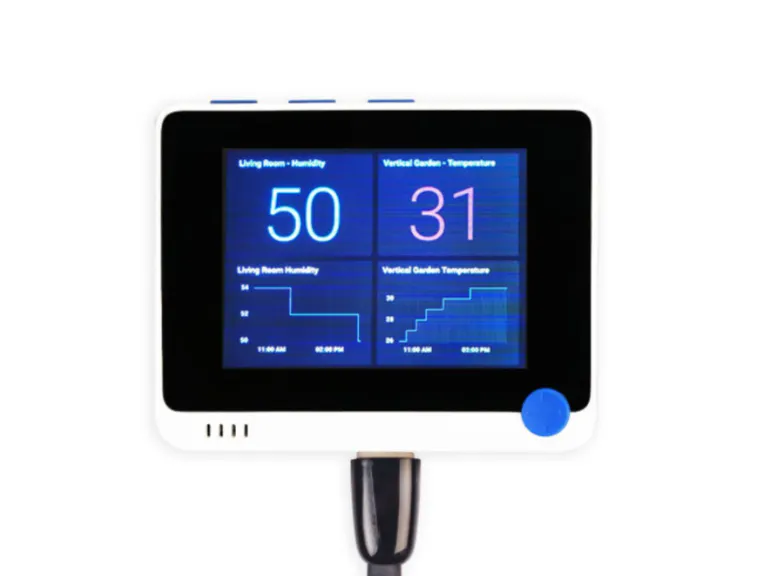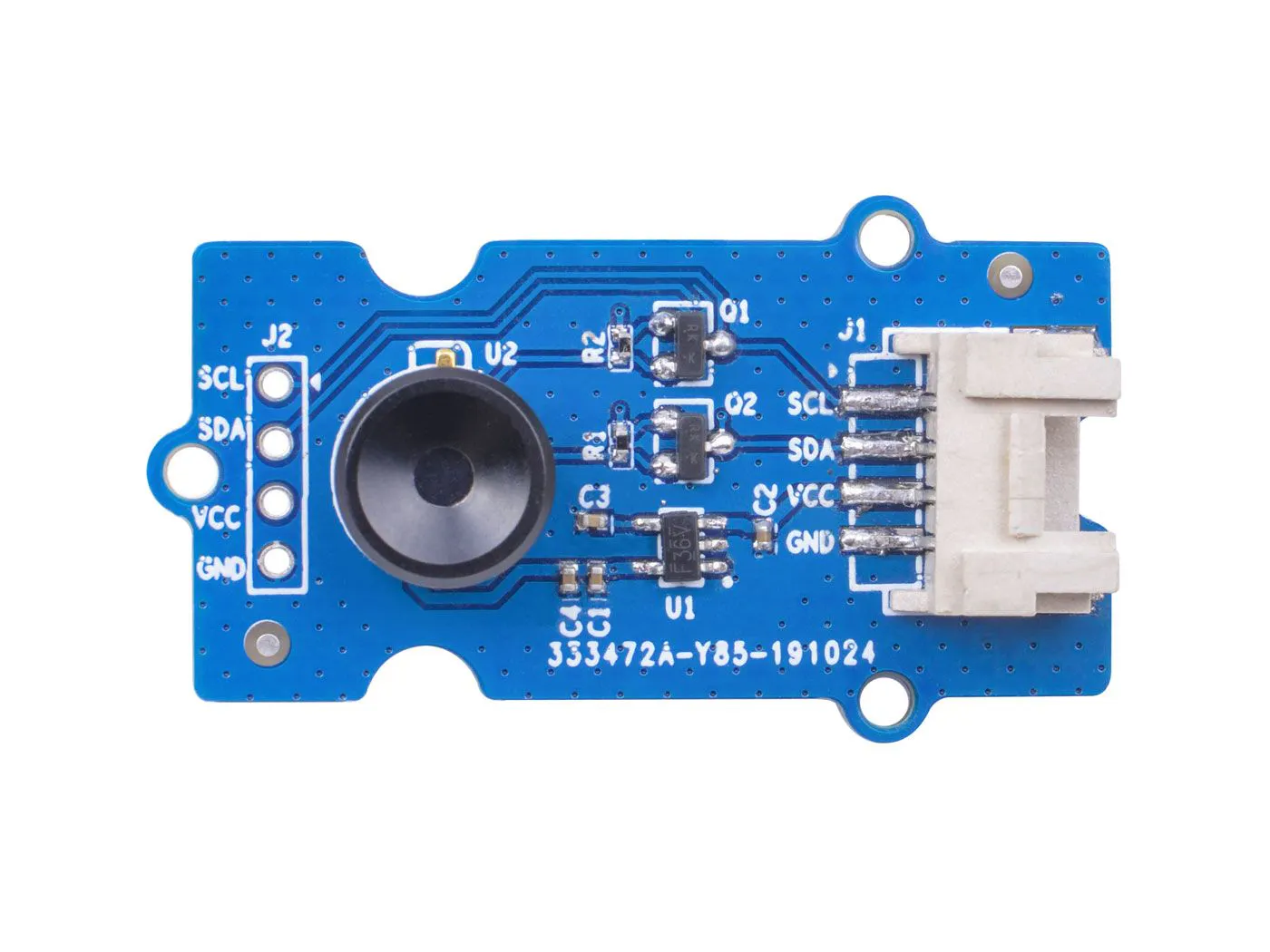# AEye: The artificial eye for your kitchen

AEye can be able to detect when your stove has been left unattended using Wio Terminal and thermal camera.

BeginnerFull instructions provided20 hours788## Things used in this project

### Hardware componentsSeeed Studio Wio Terminal
×1Seeed Studio Grove - Thermal Imaging Camera / IR Array MLX90640 110 degree
×1
 Seeed Studio Wio-Terminal-Chassis
×1
 Seeed Studio SenseCAP M2
×1

### Software apps and online servicesArduino IDEEdge Impulse StudioAmazon Web Services AWS LambdaAmazon Web Services AWS IAMAmazon Web Services AWS DynamoDB

## Code

### Data Capture

Arduino
```#include <Wire.h>
#include <SPI.h>
#include "TFT_eSPI.h"
#include "MLX90640_API.h"
#include "MLX9064X_I2C_Driver.h"
#include <Seeed_FS.h>
#include "SD/Seeed_SD.h"

#define TA_SHIFT 8 //Default shift for MLX90640 in open air

paramsMLX90640 mlx90640;

TFT_eSPI tft;
TFT_eSprite spr = TFT_eSprite(&tft);

static float mlx90640To;
char filename;
int  file_count = 500;

void readFile(fs::FS& fs, const char* path) {
Serial.println(path);
File file = fs.open(path);

if (!file) {
Serial.println("Failed to open file for reading");
return;
}

while (file.available()) {
}
file.close();
}

void writeFile(fs::FS& fs, const char* path, String data) {
Serial.print("Writing file: ");
Serial.println(path);
File file = fs.open(path, FILE_WRITE);
if (!file) {
Serial.println("Failed to open file for writing");
return;
}

// write in chunks of 512 bytes
for ( int i = 0; i < data.length(); i = i + 512) {
if (!file.print(data.substring(i, i + 512))) {
Serial.println("Write failed");
}
}

file.close();
}

void deleteFile(fs::FS& fs, const char* path) {
Serial.print("Deleting file: ");
Serial.println(path);
if (fs.remove(path)) {
Serial.println("File deleted");
} else {
Serial.println("Delete failed");
}
}

void setup()
{
pinMode(WIO_KEY_A, INPUT_PULLUP);
pinMode(WIO_KEY_B, INPUT_PULLUP);
pinMode(WIO_KEY_C, INPUT_PULLUP);
pinMode(LED_BUILTIN, OUTPUT);
digitalWrite(LED_BUILTIN, LOW);

Wire.begin();
Wire.setClock(400000); //Increase I2C clock speed to 400kHz

Serial.begin(115200);
delay(1000);
// while (!Serial); //Wait for user to open terminal

if (isConnected() == false)
{
while (1);
}
Serial.println("MLX90640 online!");

//Get device parameters - We only have to do this once
int status;
uint16_t eeMLX90640;
if (status != 0)

status = MLX90640_ExtractParameters(eeMLX90640, &mlx90640);
if (status != 0)
Serial.println("Parameter extraction failed");

//Once params are extracted, we can release eeMLX90640 array
tft.begin();
tft.setRotation(3);
Getabcd();
tft.fillScreen(TFT_BLACK);

while (!SD.begin(SDCARD_SS_PIN, SDCARD_SPI)) {
Serial.println("Card Mount Failed");
return;
}

uint8_t cardType = SD.cardType();

if (cardType == CARD_NONE) {
Serial.println("No SD card attached");
return;
}

pinMode(WIO_BUZZER, OUTPUT);
digitalWrite(LED_BUILTIN, HIGH);
}

void loop()
{
for (byte x = 0 ; x < 2 ; x++) //Read both subpages
{
uint16_t mlx90640Frame;
if (status < 0)
{
Serial.print("GetFrame Error: ");
Serial.println(status);
}

float vdd = MLX90640_GetVdd(mlx90640Frame, &mlx90640);
float Ta = MLX90640_GetTa(mlx90640Frame, &mlx90640);

float tr = Ta - TA_SHIFT; //Reflected temperature based on the sensor ambient temperature
float emissivity = 0.95;

MLX90640_CalculateTo(mlx90640Frame, &mlx90640, emissivity, tr, mlx90640To);
}

uint32_t color;
uint8_t label_id = 0;
//A - Stove with human, B - Just stove, C - Stove off
Serial.println("Stove with human");
label_id = 1;

}
else if (digitalRead(WIO_KEY_B) == LOW) {
Serial.println("Just stove");
label_id = 2;
}
else if (digitalRead(WIO_KEY_C) == LOW) {
Serial.println("Stove off");
label_id = 3;
}

String data;
for (uint8_t x = 0; x < 32; x++) {
for (uint8_t y = 0; y < 24; y++) {
if (x == 0 && y == 0) {
data = mlx90640To[24 * x + y];
} else {
data = data + "," +  mlx90640To[24 * x + y];
}

float val = mlx90640To[32 * (23 - y) + x];

if (val > 99.99) val = 99.99;

// if (val > 32.0) {
//   color = TFT_MAGENTA;
// } else if (val > 29.0) {
//   color = TFT_RED;
// } else if (val > 26.0) {
//   color = TFT_YELLOW;
// } else if (val > 20.0) {
//   color = TFT_GREENYELLOW;
// } else if (val > 17.0) {
//   color = TFT_GREEN;
// } else if (val > 10.0) {
//   color = TFT_CYAN;
// } else {
//   color = TFT_BLUE;
// }

tft.fillRect(x * 10, y * 10, 10, 10, GetColor(val));
}
}

if (label_id > 0) {
analogWrite(WIO_BUZZER, 128);
Serial.println(data);
writeFile(SD, filename, data);
file_count++;
}
delay(500);

if (label_id > 0) {
analogWrite(WIO_BUZZER, 0);
}
}

//Returns true if the MLX90640 is detected on the I2C bus
boolean isConnected()
{
if (Wire.endTransmission() != 0)
return (false); //Sensor did not ACK
return (true);
}

// variables for interpolated colors
byte red, green, blue;
uint16_t MinTemp = 25;
uint16_t MaxTemp = 38;
float  a, b, c, d;

// function to get the cutoff points in the temp vs RGB graph
void Getabcd() {

a = MinTemp + (MaxTemp - MinTemp) * 0.2121;
b = MinTemp + (MaxTemp - MinTemp) * 0.3182;
c = MinTemp + (MaxTemp - MinTemp) * 0.4242;
d = MinTemp + (MaxTemp - MinTemp) * 0.8182;

}

uint16_t GetColor(float val) {

red = constrain(255.0 / (c - b) * val - ((b * 255.0) / (c - b)), 0, 255);

if ((val > MinTemp) & (val < a)) {
green = constrain(255.0 / (a - MinTemp) * val - (255.0 * MinTemp) / (a - MinTemp), 0, 255);
}
else if ((val >= a) & (val <= c)) {
green = 255;
}
else if (val > c) {
green = constrain(255.0 / (c - d) * val - (d * 255.0) / (c - d), 0, 255);
}
else if ((val > d) | (val < a)) {
green = 0;
}

if (val <= b) {
blue = constrain(255.0 / (a - b) * val - (255.0 * b) / (a - b), 0, 255);
}
else if ((val > b) & (val <= d)) {
blue = 0;
}
else if (val > d) {
blue = constrain(240.0 / (MaxTemp - d) * val - (d * 240.0) / (MaxTemp - d), 0, 240);
}

// use the displays color mapping function to get 5-6-5 color palet (R=5 bits, G=6 bits, B-5 bits)
return spr.color565(red, green, blue);

}
```

### AEye_Inference_lora

Arduino
```#include <Wire.h>
#include <SPI.h>
#include "TFT_eSPI.h"
#include "MLX90640_API.h"
#include "MLX9064X_I2C_Driver.h"
#include <AEye_inferencing.h>
#include "gps.h"
#include "SoftwareSerial2.h"
#include <Arduino.h>

//TFT_eSPI tft;
#include "fonts.h"
#define X_OFFSET 2
#define Y_OFFSET 0
#define X_SIZE 80
#define Y_SIZE 20
#define R_SIZE 4
#define BOX_SPACING 2

#define TFT_GRAY 0b1010010100010000
#define TFT_GRAY10 0b0100001100001000
#define TFT_GRAY20 0b0010000110000100

#define HIST_X_OFFSET 2
#define HIST_Y_OFFSET 75
#define HIST_X_SIZE 315
#define HIST_X_TXTSIZE X_SIZE - 3
#define HIST_Y_SIZE 160
#define HIST_X_BAR_OFFSET 50
#define HIST_X_BAR_SPACE 2

#define AWS_PUSH_INTERVAL_IN_SECONDS 60
SoftwareSerial1 softSerial2(40, 41);

String label = "Background";
float score = 0.0;
long lastSent = 0;
long lastMoved = 0;
int position = 0;

float features;

//float data;

int raw_feature_get_data(size_t offset, size_t length, float *out_ptr) {
memcpy(out_ptr, features + offset, length * sizeof(float));
return 0;
}

#define TA_SHIFT 8 //Default shift for MLX90640 in open air

paramsMLX90640 mlx90640;
TFT_eSPI tft;
TFT_eSprite spr = TFT_eSprite(&tft); // Sprite

static float mlx90640To;

// variables for LORA
char str = {0};
char str_devEui = {0};
char str_appEvi = {0};

static int P_temp, P_humi, P_is_exist = 1, P_is_join = 1;
static char recv_buf;
static bool is_exist = false;
static bool is_join = false;
static int led = 0;
static bool Lora_is_busy = false;
static int temp = 0;
static int humi = 0;
static int switch_UI = 0;
static int init_ui = 0;

enum e_module_Response_Result{
MODULE_IDLE,
MODULE_RECEIVING,
MODULE_TIMEOUT,
MODULE_ACK_SUCCESS,
};

enum e_module_AT_Cmd{
AT_OK,
AT_ID,
AT_MODE,
AT_DR,
//AT_RATE,
AT_CH,
AT_KEY,
AT_CLASS,
AT_PORT,
//AT_LW,
AT_JOIN,
AT_CMSGHEX,
AT_TIMOUT
};

int module_AT_Cmd = 0;
typedef struct s_E5_Module_Cmd{
char p_ack;
int timeout_ms;
char p_cmd;
} E5_Module_Cmd_t;

E5_Module_Cmd_t E5_Module_Cmd[] ={
{"+AT: OK", 1000, "AT\r\n"},
{"+ID: AppEui", 1000, "AT+ID\r\n"},
{"+MODE", 1000, "AT+MODE=LWOTAA\r\n"},
{"+DR", 1000, "AT+DR=US915\r\n"},
//{"+RATE", 1000, "AT+DR=0\r\n"},
{"CH", 1000, "AT+CH=NUM,8-15\r\n"},
{"+KEY: APPKEY", 1000, "AT+KEY=APPKEY,\"2B7E151628AED2A6ABF7158809CF4F3D\"\r\n"},
{"+CLASS", 1000, "AT+CLASS=A\r\n"},
{"+PORT", 1000, "AT+PORT=2\r\n"},
//{"+LW", 1000, "AT+LW=LEN\r\n"},
{"Done", 10000, "AT+JOIN\r\n"},
{"Done", 30000, ""},
};

static int at_send_check_response(char *p_ack, int timeout_ms, char *p_cmd, ...){
int ch;
int num = 0;
int index = 0;
int startMillis = 0;
va_list args;
memset(recv_buf, 0, sizeof(recv_buf));
va_start(args, p_cmd);
softSerial2.printf(p_cmd, args);
Serial.printf(p_cmd, args);
va_end(args);

startMillis = millis();

if (p_ack == NULL){
return 0;
}
do{
while (softSerial2.available() > 0)
{
recv_buf[index++] = ch;
Serial.print((char)ch);
delay(2);
}

if (strstr(recv_buf, p_ack) != NULL){
return 1;
}

} while (millis() - startMillis < timeout_ms);
return 0;
}

static int at_send_check_response_flag(int timeout_ms, char *p_cmd, ...){
if (Lora_is_busy == true){
return 0;
}
Lora_is_busy = true;
//  Lora_Ack_timeout = timeout_ms;
va_list args;
memset(recv_buf, 0, sizeof(recv_buf));
va_start(args, p_cmd);
softSerial2.printf(p_cmd, args);
Serial.printf(p_cmd, args);
va_end(args);
return 1;
}

static int check_message_response(){
static bool init_flag = false;
static int startMillis = 0;
static int index = 0;
e_module_Response_Result result = MODULE_ACK_SUCCESS;
int ch;
int num = 0;
if (Lora_is_busy == true){
if (init_flag == false){
startMillis = millis();
init_flag = true;
index = 0;
memset(recv_buf, 0, sizeof(recv_buf));
Serial.println("Cmd Start......");
}
Lora_is_busy = false;
init_flag = false;
while (softSerial2.available() > 0){
recv_buf[index++] = ch;
Serial.print((char)ch);
delay(2);
}

if (strstr(recv_buf, E5_Module_Cmd[module_AT_Cmd].p_ack) != NULL){
//          is_join = true;
return result;
}

if (millis() - startMillis >= E5_Module_Cmd[module_AT_Cmd].timeout_ms){
Serial.println("Cmd Timeout......");
return MODULE_TIMEOUT;
}
Lora_is_busy = true;
init_flag = true;
return MODULE_RECEIVING;
}
return MODULE_IDLE;
}

void print_EVI(){
Serial.println("+++++++++++ Devicee Info ++++++++++");
Serial.printf("Device EUI %s \n",str_devEui);
Serial.printf("App EUI %s \n",str_appEvi);
Serial.printf("App Key 2B7E151628AED2A6ABF7158809CF4F3D \n");
Serial.println("+++++++++++++++++++++++++++++++++++");
}
void display_EVI(){
//  static int init_ui = 0;
if (init_ui == 0){
init_ui = 1;

tft.setTextSize(1);
tft.setFreeFont(FM9);
tft.setTextColor(TFT_WHITE);
tft.drawString("Device", 5, 40, GFXFF);
tft.drawRoundRect(HIST_X_OFFSET, HIST_Y_OFFSET - 20, HIST_X_SIZE, HIST_Y_SIZE + 20, R_SIZE, TFT_WHITE);

tft.setFreeFont(FSSO9);
tft.setTextColor(TFT_BLUE);
tft.drawString("LoRaWAN", HIST_X_OFFSET + 10, HIST_Y_OFFSET , GFXFF);
tft.setFreeFont(FM9);
tft.setTextColor(TFT_WHITE);
tft.fillRect(HIST_X_OFFSET + 10, HIST_Y_OFFSET + 25, 300, 15, TFT_BLACK);
strcpy(str, "DevEui:");
strcat(str, str_devEui);
tft.drawString(str, HIST_X_OFFSET + 10, HIST_Y_OFFSET + 25, GFXFF);
memset(str, 0, sizeof(str));
tft.fillRect(HIST_X_OFFSET + 10, HIST_Y_OFFSET + 45, 300, 15, TFT_BLACK);
strcpy(str, "AppEui:");
strcat(str, str_appEvi);
tft.drawString(str, HIST_X_OFFSET + 10, HIST_Y_OFFSET + 45, GFXFF);
tft.drawString("AppKey:", HIST_X_OFFSET + 10, HIST_Y_OFFSET + 65, GFXFF);
tft.drawString("\"", HIST_X_OFFSET + 10, HIST_Y_OFFSET + 65, GFXFF);
tft.drawString("2B7E151628AED2A6AB", HIST_X_OFFSET + 88, HIST_Y_OFFSET + 65, GFXFF);
tft.drawString("F7158809CF4F3D", HIST_X_OFFSET + 88, HIST_Y_OFFSET + 85, GFXFF);

}
}

void setup() {
lastSent = millis();
pinMode(LED_BUILTIN, OUTPUT);
digitalWrite(LED_BUILTIN, LOW);

Wire.begin();
Wire.setClock(400000);  //Increase I2C clock speed to 400kHz

Serial.begin(115200);
delay(1000);
// while (!Serial); //Wait for user to open terminal

if (isConnected() == false) {
while (1)
;
}
Serial.println("MLX90640 online!");

//Get device parameters - We only have to do this once
int status;
uint16_t eeMLX90640;
if (status != 0)

status = MLX90640_ExtractParameters(eeMLX90640, &mlx90640);
if (status != 0)
Serial.println("Parameter extraction failed");

//Once params are extracted, we can release eeMLX90640 array
tft.begin();
tft.setRotation(3);

tft.setTextSize(2);
tft.setTextColor(TFT_WHITE);
tft.fillScreen(TFT_BLACK);
tft.drawString("Camper is initializing...", 20, 120);
tft.fillScreen(TFT_BLACK);

//connect to cloud

pinMode(WIO_BUZZER, OUTPUT);
digitalWrite(LED_BUILTIN, HIGH);
pinMode(WIO_5S_LEFT, INPUT_PULLUP);
pinMode(WIO_5S_RIGHT, INPUT_PULLUP);

GpsSerialInit();

softSerial2.begin(9600);
softSerial2.listen();
while (!softSerial2);

delay(5000);
}

int pLabel = 0; // 0-backgroud , 1- human, 2- animal
int pScore = 0;

void loop() {

tft.fillScreen(TFT_BLACK);
print_EVI();
switch_UI = -1;

}

switch_UI = 0;
}

if(switch_UI == -1){
display_EVI();

}

if ((millis()-lastRead) > 60 * 1000){
GpsstopListening();
delay(100);
GpsSerialInit();

}

GetGpsInfoPolling();
UpdateGpsInfo();
int r = check_message_response();
//Serial.printf("Loop - %d \n", r);
switch (r){
case MODULE_IDLE:
if (module_AT_Cmd <= AT_PORT){
//if (module_AT_Cmd <= AT_LW){
at_send_check_response_flag(E5_Module_Cmd[module_AT_Cmd].timeout_ms, E5_Module_Cmd[module_AT_Cmd].p_cmd);
Serial.print("module_AT_Cmd = ");
Serial.println(module_AT_Cmd);
}
else if (is_join == true){
if ((millis() - lastSent)  > 30 * 1000)
{
int lat = (int)(Lat* (-0.01));
int lon = (int)(Lng*0.01);
Serial.printf("\$\$\$\$\$\$  Sending Data %d %d %d %d\n",pLabel,pScore, lat, lon);
lastSent = millis();
char cmd;
int l1 = 4141;
module_AT_Cmd = AT_CMSGHEX;
sprintf(cmd, "AT+CMSGHEX=\"%02X%02X%08X%08X\"\r\n", byte(pLabel), byte(pScore), lat,lon);
at_send_check_response_flag(E5_Module_Cmd[module_AT_Cmd].timeout_ms, cmd);

}
}
else if (is_join == false){
Serial.printf("Not Connected. Trying to join... \n");
if ((millis() - lastSent) > 5 * 1000){
Serial.println("Send Jion");
lastSent = millis();
module_AT_Cmd = AT_JOIN;
at_send_check_response_flag(E5_Module_Cmd[module_AT_Cmd].timeout_ms, E5_Module_Cmd[module_AT_Cmd].p_cmd);
}
}

break;
case MODULE_RECEIVING:
break;
case MODULE_TIMEOUT:
is_exist = false;
is_join  = false;
module_AT_Cmd = AT_OK;
Serial.println("MODULE_TIMEOUT");
break;
case MODULE_ACK_SUCCESS:
is_exist = true;

switch (module_AT_Cmd){
case AT_JOIN:
if (strstr(recv_buf, "Network joined") != NULL){
is_join = true;
}
else{
is_join = false;
}
break;

case AT_ID:
int j = 0;
char *p_start = NULL;
p_start = strstr(recv_buf, "DevEui");
sscanf(p_start, "DevEui, %23s,", &str);//&E5_Module_Data.DevEui);
j = 0;
for (int i = 0; i < 16; i++, j++){
if ((i != 0) && (i % 2 == 0)){
j += 1;
}
str_devEui[i] = str[j];
}
Serial.println(str_devEui);
p_start = strstr(recv_buf, "AppEui");
sscanf(p_start, "AppEui, %23s,", &str);//&E5_Module_Data.AppEui);
j = 0;
for (int i = 0; i < 16; i++, j++){
if ((i != 0) && (i % 2 == 0)){
j += 1;
}
str_appEvi[i] = str[j];
}
Serial.println(str_appEvi);
init_ui = 0;
break;

}

if (module_AT_Cmd <= AT_PORT){
//if (module_AT_Cmd <= AT_LW){
module_AT_Cmd++;
}
break;
}

for (byte x = 0; x < 2; x++)  //Read both subpages
{
uint16_t mlx90640Frame;
if (status < 0) {
Serial.print("GetFrame Error: ");
Serial.println(status);
}

float vdd = MLX90640_GetVdd(mlx90640Frame, &mlx90640);
float Ta = MLX90640_GetTa(mlx90640Frame, &mlx90640);

float tr = Ta - TA_SHIFT;  //Reflected temperature based on the sensor ambient temperature
float emissivity = 0.95;

MLX90640_CalculateTo(mlx90640Frame, &mlx90640, emissivity, tr, mlx90640To);
}

uint32_t color;
uint8_t label_id = 0;
//A - Stove with human , B- Just stove , C - Stove off

String data;
int counter = 0;

for (uint8_t x = 0; x < 32; x++) {
for (uint8_t y = 0; y < 24; y++) {
if (x == 0 && y == 0) {
data = mlx90640To[24 * x + y];
} else {
data = data + "," + mlx90640To[24 * x + y];
}
features[counter++] = mlx90640To[24 * x + y];

float val = mlx90640To[32 * (23 - y) + x];

if (val > 99.99) val = 99.99;

if (val > 32.0) {
color = TFT_MAGENTA;
} else if (val > 29.0) {
color = TFT_RED;
} else if (val > 26.0) {
color = TFT_YELLOW;
} else if (val > 20.0) {
color = TFT_GREENYELLOW;
} else if (val > 17.0) {
color = TFT_GREEN;
} else if (val > 10.0) {
color = TFT_CYAN;
} else {
color = TFT_BLUE;
}

if(switch_UI == 0)
{
tft.fillRect(x * 10, y * 10, 10, 10, color);
}
}
}
//delay(500);
//ei_printf("Counter %d \n", counter);
//ei_printf("Edge Impulse standalone inferencing (Arduino)\n");

if (sizeof(features) / sizeof(float) != EI_CLASSIFIER_DSP_INPUT_FRAME_SIZE) {
ei_printf("The size of your 'features' array is not correct. Expected %lu items, but had %lu\n",
EI_CLASSIFIER_DSP_INPUT_FRAME_SIZE, sizeof(features) / sizeof(float));
delay(1000);
return;
}
ei_impulse_result_t result = { 0 };

// the features are stored into flash, and we don't want to load everything into RAM
signal_t features_signal;
features_signal.total_length = sizeof(features) / sizeof(features);
features_signal.get_data = &raw_feature_get_data;

// invoke the impulse
EI_IMPULSE_ERROR res = run_classifier(&features_signal, &result, false /* debug */);
//ei_printf("run_classifier returned: %d\n", res);

if (res != 0) return;

// print the predictions
score = 0.0;
//ei_printf("Predictions ");

for (size_t ix = 0; ix < EI_CLASSIFIER_LABEL_COUNT; ix++) {
//ei_printf("    %s: %.5f\n", result.classification[ix].label, result.classification[ix].value);
if ( result.classification[ix].value >= score) {
score = result.classification[ix].value;
label = result.classification[ix].label;
}
}

pScore = (int) (score*100);

if(label == "nostove"){
pLabel = 0;
}else if(label == "stovewithhuman"){
pLabel = 1;
}else{
pLabel = 2;
}

ei_printf("Predicted label = %s with score %f\n", label.c_str(), score);

}

//Returns true if the MLX90640 is detected on the I2C bus
boolean isConnected() {
if (Wire.endTransmission() != 0)
return (false);  //Sensor did not ACK
return (true);
}

void ei_printf(const char *format, ...) {
static char print_buf = { 0 };

va_list args;
va_start(args, format);
int r = vsnprintf(print_buf, sizeof(print_buf), format, args);
va_end(args);

if (r > 0) {
Serial.write(print_buf);
}
}
```

### Lambda Function

Python
```import json
import boto3
from botocore.exceptions import ClientError
import time

def lambda_handler(event, context):
pinpoint_client = boto3.client('pinpoint')
dynamoClient = boto3.client('dynamodb')

origination_number = "Your aws long code"
message_type = "TRANSACTIONAL"

try:
if data["label"] == 2:

response = dynamoClient.get_item(TableName ="wio_terminal_stove_config",Key={"id": {"S" :"LAST_TEXT_SENT"}} )
past = (int) (response['Item']['value']['S'])
now = (int) (time.time())
diff = now - past
print("diffrence", diff)
# don't send text within 15
if diff > 15*60:

response = pinpoint_client.send_messages(
ApplicationId=app_id,
MessageRequest={
'MessageConfiguration': {
'SMSMessage': {
'Body': message,
'MessageType': message_type,
'OriginationNumber': origination_number}}})

response = dynamoClient.update_item(
TableName ="wio_terminal_stove_config",
Key={"id": {"S" :"LAST_TEXT_SENT"}},
'value': {"Value":{"S":str(now)}},
}
)
#print(response)

print("data updated successfully")
else:
print("data updated failed!!!")

except ClientError:
logger.exception("Couldn't send message.")
raise

return {
'statusCode': 200,
'body': json.dumps('Hello from Lambda!')
}
```

## Credits

### Sashrika Das

0 projects • 1 follower